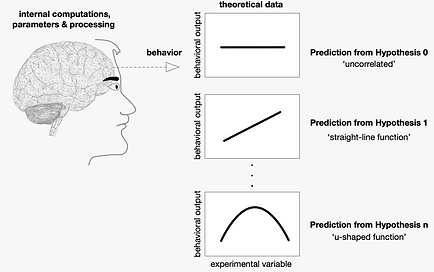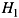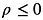top of page

#### A Beginners Guide to Statistical Hypothesis Testing

• What are scientific hypotheses and models?

• What is the logic underlying statistical hypothesis testing?

• How are statistical hypothesis tests computed and interpreted?

• Are there logical flaws in the standard procedure for statistical hypothesis testing?

What are scientific hypotheses and models?

Our goal in analyzing data is usually to compare hypotheses. In the neural and behavioral sciences, we want to know if experimental data contain evidence to support one hypothesis regarding the causes of behavior over one or more alternative hypotheses regarding the same behavior.

Hypotheses are word-based descriptions of the causal mechanisms that are posited to account for an aspect of behavior, such as a behavioral change following stroke, or the details of the behavioral (motor, cognitive) sequelae of Parkinson’s disease. Models translate those word-based descriptions into the mathematical relationships that we ultimately use when comparing hypotheses with experimental data.

The models derived from each hypothesis will be used to make quantitative predictions about the values of experimental data, and statistical testing will consist of computations based on those data and predictions. In Figure 1, we’ve suggested a range of hypotheses to describe some particular behavior, and the changes in that behavior that might result from an experimental intervention. For example, each model might represent they way different hypotheses predict recovery from stroke (‘behavioral output’) under the influence of increasing dosages of a certain drug (‘experimental variable’).

What is the logic underlying statistical hypothesis testing?

There are several steps to the logic of statistical hypothesis testing (which is a blend of ideas derived from the theories of Fisher and Neyman):

• The first step is to define the hypothesis you want to test: the statistical ‘null hypothesis’, labeled      . This hypothesis will always predict ‘no effect’ from experimental manipulations
• Generate the specific predictions about data based on the details of your experiment. In the drug dosage example above, the null hypothesis is that the drug has no effect on behavior (and therefore no effect at any dosage), which corresponds to the flat line  (zero correlation) prediction

• compute the probability distribution over possible datasets (correlation statistics) from     and the criterion statistic corresponding to your alpha-criterion (in Fig 2, the edges of the tail-area of the distribution that cover                of the probability mass,        )

• collect data (shown in Figure 3), and compute the data correlation (       )

• compute the p-value associated with the data (contingent on the truth of the null hypothesis), and compare it to the criterion statistic / alpha-level. The criterion statistic (criterion correlation, rcrit, in this example) is a vertical red line in Figure 2

• The p-value is the sum of the probabilities corresponding to correlation statistics that are more extreme,                 , than the observed correlation (                ). In Figure 4 the p-value is the grey shaded probability mass.

• if the p-value is less than the    -criterion (usually               ), or equivalently if the observed statistic (here, the data correlation) is more extreme than the criterion statistic,                    , the null hypothesis is rejected.

Why are statistical hypothesis tests structured in this way, with a sampling distribution (Figs. 2, 4) and p-values?

The goal is to reject the null hypothesis, which posits no effect present in the data, by assessing whether there is a reliable effect present in your data.

In this example, we interpret data correlations that are larger than the critical value of the statistic as evidence of a reliable difference from the predictions of the null hypothesis, because that data correlation is not supposed to happen often when the null hypothesis is correct.

How are statistical hypothesis tests computed and interpreted?

For the correlation example we are exploring here, the hypothesis test is based on the data correlation:

and this value is compared to the critical value that you compute based on the    -criterion.

Most statistical packages will have built-in statistical tests to perform these computations and produce the desired p-value. In Matlab, this is:

x=[-10:10]'; d=[-10:10]'+9*randn(21,1);

[r p]=corr(x,d)

If you wanted to re-create Figures 2 through 4 and compute correlations and p-values from scratch, you could write:

rdat=sum((x-mean(x)).*(d-mean(d)))/(sqrt(sum((x-mean(x)).^2))*sqrt(sum((d-mean(d)).^2))); %data correlation

figure; plot(x,d,'ko','MarkerSize',10,'MarkerFaceColor',[.2 .3 .4]); axis([-25 25 -25 25]) %plot of data Fig2

Nr=5001; %number of correlations in the discretized probability distribution over correlation

r=linspace(-1,1,Nr+2); r=r(2:end-1);

zlist=.5*log((1+r)./(1-r))*sqrt(length(d)-3); zdat=.5*log((1+rdat)/(1-rdat))*sqrt(length(d)-3);

plist=normpdf(zlist); %probability distribution over correlation coefficients

icrit=[find(zlist>-1.96,1)-1; find(zlist>1.96,1)]; %index corresponding to critical values of r

pcheck=2*normcdf(zlist(icrit(1))); %verify that the alpha-level defined by the criterion r-value is 0.05

idat=[find(r<-abs(rdat),1,'last') find(r>abs(rdat),1)]; %index for +/- rdat values

pval=normcdf(-abs(zdat))+(1-normcdf(abs(zdat))); %the p-value is the sum of probabilities in BOTH tails

figure; hold on %figure3 showing the critical values of r, and the shaded alpha=0.05 tail-areas

iz=[1 icrit(1); icrit(2) Nr];

for irow=1:2,

for inow=iz(irow,1):iz(irow,2),

plot(r(inow)*[1 1],[0 plist(inow)],'-','Color','k'); end, end

plot(r(icrit(1))*[1 1],[0 plist(icrit(1))],'-','LineWidth',3,'Color',[.35 0 0])

plot(r(icrit(2))*[1 1],[0 plist(icrit(2))],'-','LineWidth',3,'Color',[.35 0 0])

plot(r,plist,'k.');

figure; hold on %figure4 showing the +/- values of rdat, and the shaded tail-areas defining the p-value

iz=[1 idat(1); idat(2) Nr];

for irow=1:2,

for inow=iz(irow,1):iz(irow,2),

plot(r(inow)*[1 1],[0 plist(inow)],'-','Color',.7*[1 1 1]); end, end

plot(r(idat(2)-1),0,'ko','MarkerSize',8,'MarkerFaceColor',[.2 .3 .4])

plot(r(icrit(1))*[1 1],[0 plist(icrit(1))],'-','LineWidth',2,'Color',[.35 0 0])

plot(r(icrit(2))*[1 1],[0 plist(icrit(2))],'-','LineWidth',2,'Color',[.35 0 0])

plot(r,plist,'k.');

• Fig 4 shows the interpretation of the result. When the correlation (                ) is larger (in absolute value) than the criterion statistic (                ), then the p-value will be p< 0.05 and ‘the null hypothesis is rejected’.

• Contrariwise, if the absolute value of the data correlation had been less than the criterion statistic (and therefore p-value > 0.05), we ‘fail to reject the null hypothesis’.

Rejecting the null hypothesis means that we have concluded that the null hypothesis is incorrect. This is straightforward as far as it goes: the data are outside the acceptable range of prediction, and so we conclude that the assumption upon which that prediction was made is incorrect.

When we ‘fail to reject’ the situation is less straightforward. Your natural inclination will be to interpret this as a situation where we have found evidence to accept the null hypothesis. This inference is strictly forbidden. You can never never never, under the logic of statistical hypothesis testing, find direct statistical evidence supporting your null hypothesis. Instead, your goal is to reject all competing hypotheses so that there is only one alternative left standing. If there is only one possible explanation, then it must be the correct explanation.

What is the difference between a one- and two-tailed hypothesis test?

Most hypothesis tests are two-tailed. This means that you would reject the null hypothesis test if the data deviate from the canonical prediction of the null hypothesis (examples from clinical trials might be: (a) no difference in recovery between control and patient groups, (b) no correlation between drug dosage and recovery). The latter is shown graphically in the upper plot of Figure 5.

The null hypothesis will be rejected if it strays too far from the canonical prediction of the null hypothesis in either direction, positive or negative.

This is likely the most common type of null hypothesis test because no alternative hypothesis (   ) need be defined

However, if you have a prediction for the direction of deviation from the null hypothesis that would be consistent with an alternative to the null hypothesis (alternative hypothesis,     ), you use a one-sided hypothesis test.

• If the viable alternative hypothesis predicts:

1.    : a positive mean value (r > 0)

• the null hypothesis is treated as if it includes either no difference, or also a possible negative difference

2.    : a negative mean value (r > 0)

• the null hypothesis is treated as if it includes either no difference, or also a possible positive difference

• Option 2 is shown in the lower panel of Fig. 5. ​

• This hypothesis test has no capacity to detect a positive deviation from zero.
• However, it has an easier time finding a negative deviation from zero, because the rejection region in the negative tail is now larger.

Are there logical flaws in the standard procedure for statistical hypothesis testing?

The logic of hypothesis testing was described above in a way that will (hopefully) allow you to make some sense of the enterprise. However, if you dig a little deeper you might come across some logical oddities. If you are taking a statistics course, you might want to ask about some of the following issues:

• The overall logic of statistical hypothesis testing is a flawed version of a valid logical argument called ‘proof by contradiction’. Proof by contradiction is an argument form with a long history in both science and mathematics. You can show that a theory/hypothesis/model is incorrect by first assuming that it is true, and then based on assuming its truth you derive a falsehood. In other words, if your theory makes a prediction that is verifiably false, then the theory cannot be true.

• In a very simplified example, this is how proof by contradiction works: your friend flips a coin three times and it shows heads each time. Your friend offers to bet on the outcome of the next flip. You refuse to bet, saying (hypothesizing) that the coin must be two-headed. Your theory can be proven incorrect if data from any subsequent flip (even a single instance) show tails.

• Statistical hypothesis testing is meant to follow this logic, but misses the mark. In my coin example I chose a situation in which the theory cannot possibly be correct if you observe a certain datum (a single instance of ‘tails'). However, scientific theories are almost always of the type where no dataset is considered impossible, and therefore no dataset can disprove your theory.

• For example, the null hypothesis associated with coin-flips is always that there is no difference between the rate of heads and tails. Based on this null hypothesis, there is a non-zero probability that you could observe a dataset of all heads (or all tails), of any finite length.

• In other words, there is no dataset that would contradict the null hypothesis. To sidestep this issue, you instead reject the null hypothesis when the probability of the dataset (computed by assuming the truth of the null) is below a preset threshold value. This is not the same as proof by contradiction, because low-probability datasets are still quite possible.

• Thus, while statistical hypothesis testing is focused on rejecting the null hypothesis (you can never accept the null hypothesis), it turns out that there is a good deal of uncertainty regarding your decision to reject. In fact, the    -level that you use to set the threshold for rejecting the null also defines the rate at which you will falsely reject the null hypothesis.

• Statistical hypothesis testing is really based on a ‘measurement’ rather than a hypothesis test.

• Measurements are contingent on computing probabilities of parameter values, which in turn require that you know the correct model of the world (i.e., measuring the parameter values defined by your model). This is what happens when you use data to measure the speed of sound. You have a model you already believe, that sound will move essentially at constant speed through the air, and that the speed will be: speed = distance / time. The speed parameter is measured (estimated) with data by assuming this model is correct.

• Similarly, statistical hypothesis tests start with a measurement of the variable of interest, such as the correlation between drug dosage and behavioral response (                in the example). It then asks if that measurement seems to be ‘reliably different’ from the measurement that is most consistent with the null hypothesis (i.e., the null hypothesis is most consistent with           ). If it seems reliably different, you reject the null; this last step is an attempt to turn a measurement into an hypothesis test.

Hypothesis tests should be contingent on the probabilities of hypotheses, because they answer the question, ‘which hypothesis is most likely to be correct, based on the data?’. Unfortunately, it is not possible to compute the probabilities of hypotheses within the statistical hypothesis-testing regime. You cannot compute the probabilities of hypotheses because the logic of statistical hypothesis testing relies of an unusual definition of probability, wherein you cannot define the probabilities of everyday things like ‘the probability that it will rain tomorrow’, or ‘the probability that you are flipping a 2-headed coin’, or ‘the probability that my hypothesis is correct’.

• There are two types of statistical hypothesis test (we’ll illustrate this issue using the correlation example from above), and there is a logical inconsistency between them

• 2-tailed hypothesis tests (described above in our examples)​

•    : the true correlation is zero​,

• 1-tailed hypothesis tests will make one of two predictions

•    : the true correlation is greater than or equal to zero,

•    : the true correlation is less than or equal to zero,

A) When your null hypothesis test is two-tailed, you have a single prediction

• no difference, mean = 0, correlation = 0, etc., are all single predictions
• that prediction has highest probability in the sampling distribution

• e.g., the sampling distribution over potential correlations under the null hypothesis,     is written:
• deviations from that prediction have lower probability

• this explains the symmetric shape of the Figs. 2, 4, 5

B) When your null hypothesis test is one-tailed, you now have many predictions

• ​differences > 0, mean       ,           , etc., are all one-tailed predictions

• the sampling distribution is still singly-peaked

• the prediction at zero is still the location of the peak of the sampling distribution

• e.g., the sampling distribution over potential correlations under the null hypothesis,     , is still written:
• However, if      predicts correlations other than zero, the sampling distribution should be written differently, and also in consequence have a potentially different shape as well as a different acceptance region

• Thus, while the null hypothesis is treated as if          or          for the purposes of one tail of the rejection region defined by your    -level, the sampling distribution itself is still computed in terms of           .Fig. 4Fig. 5Fig. 3Fig. 1

Fig. 2bottom of page